# Summer Cluster on Challenges in Quantum Computation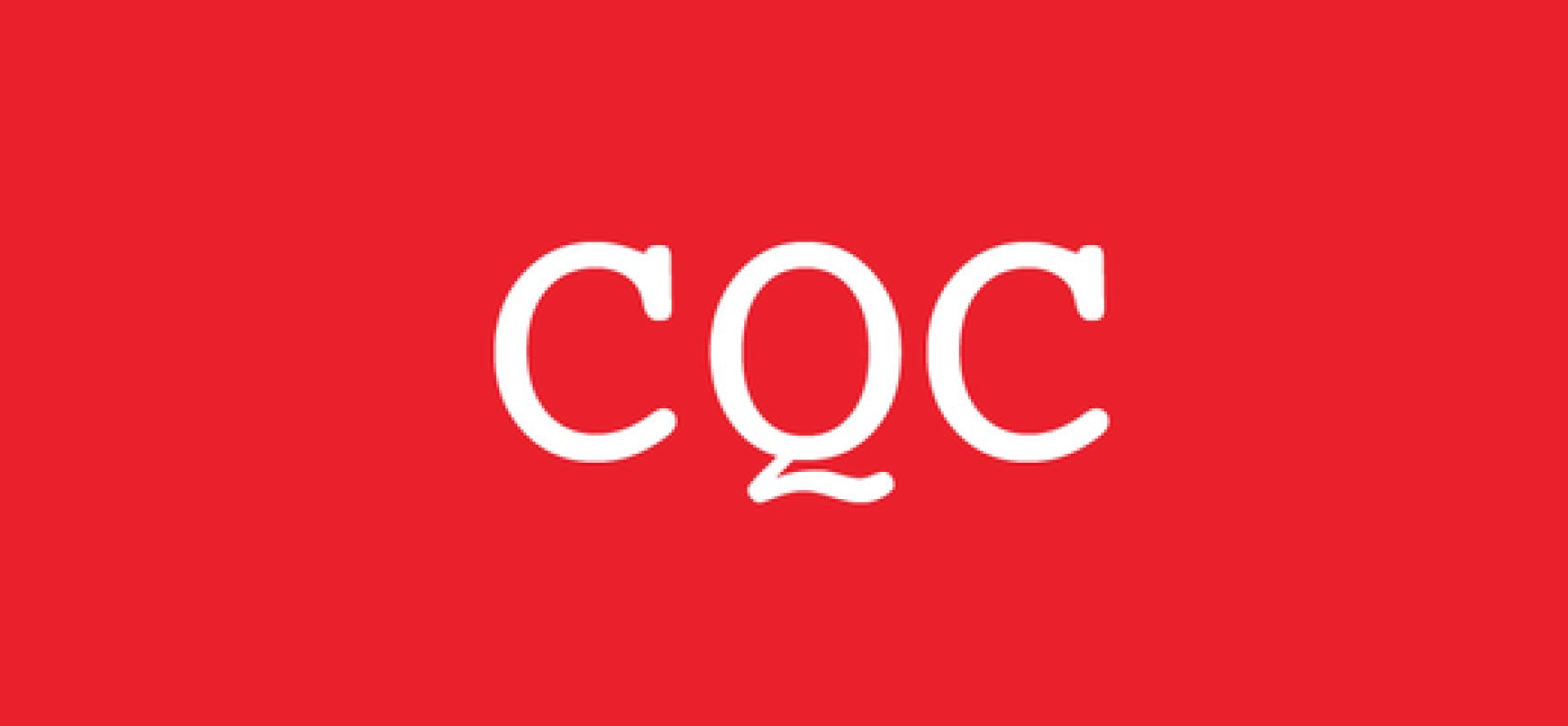In 2014, the Simons Institute for the Theory of Computing held a semester-long program on Quantum Hamiltonian Complexity. This summer, computer scientists Umesh Vazirani, Andrew Childs, Ignacio Cirac, and Thomas Vidick organized a smaller cluster with a somewhat broader focus: Challenges in Quantum Computation. This newest effort is the precursor to a full program on The Quantum Wave in Computing, scheduled for Spring 2020.

Even though only four years have passed since the first program, the field of quantum computing has undergone major changes that made this an opportune time for a second convening. Several research groups are either building or planning quantum systems that can control at least 10 and as many as 50 "qubits" (the quantum version of a bit). Though they are far from being universal computers, they have enough power to do rudimentary calculations—and even not-so-rudimentary ones (see Vignette 2). "They're actual quantum systems, not just physics experiments," said Christopher Monroe of the University of Maryland.

Both private industry and the US government have noticed, and begun investing substantial money in quantum computing. Large companies like Microsoft and Google have active research groups. Two bills are working their way through Congress that would establish a national research center for quantum computing, and they are given a reasonably good chance of passing.

The sudden explosion of corporate, governmental, and media interest in quantum computing brings both risks and rewards. One risk is that the technology will be overhyped. In fact, this has already happened to some extent. One company already claims to sell a quantum computer consisting of more than 2000 qubits, a claim that many participants at this summer's cluster treat with skepticism. There are two main issues: the quality of the qubits and the potentially limited range of applicability of its approach to computation, called quantum annealing. Effective quantum computation depends on isolating qubits or selected groups of qubits from their environment for a reasonably long period of time (the decoherence time). In particular, the decoherence time should be longer than it takes to execute the computation. The system that is the subject of the controversial claims seems to have unacceptably short decoherence times.

Beyond the hype, though, the development of actual controllable quantum systems does herald an important transition. The field of experimental quantum computing, which still seemed like a distant promise in 2014, is close to becoming a reality. For 25 years, computer scientists have been free to imagine an ideal quantum computer, with unlimited memory and perfect accuracy. The real thing will have neither of those features, and it will take a lot of work to bring these imperfect experimental devices closer to the theoretical ideal.

One reason for optimism is that the theoretical ideal for a quantum computer vastly exceeds the theoretical ideal for a classical computer, at least for certain problems. The state of an n-qubit system, if the qubits are capable of unlimited quantum entanglement, requires 2n classical bits to describe. The hope is that this statement can be reversed, and an arbitrary 2n-bit string can be encoded as a state of just n qubits. If this were literally true, even a 50-qubit quantum computer could perform computations that would tax today's most powerful supercomputers. However, there are immense practical and theoretical obstacles to realizing this "exponential speedup," and the claim has to be justified one problem at a time.

Furthermore, if true, such an exponential speedup would shake the very foundations of computer science. In 1936, the dawn of the computer age, Alan Turing defined a simple model of classical computer called a Turing machine. According to the extended Church-Turing thesis, anything that can be efficiently computed on any universal computer can also be efficiently computed on a probabilistic Turing machine. (The extra word, probabilistic, means that the Turing machine is allowed to use computations that give a correct result, say, 99.9999% of the time. This turns out to give a substantial speedup for some problems.) The extended Church-Turing Thesis has been immensely useful as a working hypothesis; the whole field of computational complexity has been predicated on the idea that a Turing machine can be used as an objective standard for all universal computers.

In 1993, Vazirani and his student Ethan Bernstein gave the first example to prove that an ideal quantum computer would violate the extended Church-Turing Thesis. That is, they found a problem ("a completely impractical problem that no one would ever want to solve," Vazirani says) and an algorithm that a quantum computer could use to solve it in exponentially faster time than a classical computer could manage. In the following year, Peter Shor of IBM took their work one step further by showing that a practical problem—in fact, not only a practical one but a linchpin of the multimillion-dollar cryptography business—could also be given an exponential quantum speedup.

Now that medium-sized quantum devices with far-from-ideal accuracy seem to be coming in the near future, perhaps the two most important questions about them are the same two questions that Vazirani, Bernstein, and Shor answered in the context of large and ideal quantum computers. First, is there anything, however impractical, that these machines can do better than classical computers? In other words, is the Church-Turing Thesis violated by a real-world machine? This would be the analogue to Vazirani's and Bernstein's theorem. Second, is there some significant or even important application that medium-sized, imperfect quantum computers can perform better than classical ones? That would be the practical analogue of Shor's theorem.

In spite of all of the hype, the answers to both of these questions are still very much unknown. The design of quantum computers and the algorithms that will run on them depend very much on the assumption that the reigning theory of quantum mechanics, which has been in place since roughly the 1930s, is an accurate theory. Of course, the validity of quantum mechanics has been tested to exquisite accuracy for systems consisting of one particle, and for very rapid interactions of a small number of particles. However, quantum computing requires us to apply the theory to a domain where it has never been adequately tested: what Vazirani calls the high-complexity regime, involving large numbers of entangled particles that remain entangled for a substantial amount of time.

Perhaps there will be no new surprises in this regime. If so, it will be good news for quantum computing. But from the historical perspective, that would be a somewhat surprising outcome. In the past, each time physicists explored a new regime, such as high velocities or small sizes and high energies, they have thought they knew what to expect, and they were wrong. They had to devise new theories (relativity theory in the first case, quantum theory in the second) to account for the new phenomena that the experiments revealed. For this reason, Vazirani argues, "The importance of quantum computing is not only in the technology but in the science. However, the science lies on the path to technology."

With so many possibilities opening up, the Summer 2018 cluster was broader in scope than the 2014 program. Some of the topics under discussion, and recent results that have attracted attention in the field, were the following:

• Hamiltonian simulation. Related title topic of the 2014 program on Quantum Hamiltonian Complexity, this has to do with the very natural problem from physics of simulating the time evolution of a quantum system under a prescribed energy functional, or Hamiltonian. Some impressive new results on a technique called qubitization were announced by András Gilyén, who had worked in collaboration with several others. (Vignette 1.)

• Quantum chemistry. In theory, this is a subset of Hamiltonian simulation, using certain prescribed Hamiltonians favored by chemists. The philosophy, however, is very different. While the Hamiltonian problem has been driven by abstract mathematical results, chemists are interested in specific real-world questions: How do catalysts work? How can we simulate reactions of molecules in excited states, or in exotic states where electrons have correlated spins? Chemists do not particularly care whether the answers come from quantum computers or classical computers. Nevertheless, many people believe that this area is where the first real-world applications of quantum computing will be found. Martin Head-Gordon of UC Berkeley and James Whitfield of Dartmouth College gave insightful talks on the problems that matter to quantum chemists. (Vignette 2.)

• Random quantum circuits. One of the first experiments with intermediate-scale quantum devices, which Google plans to conduct later in 2018, will involve simulating the output of a random quantum circuit. In essence, we are asking a quantum computer to simulate a random version of itself—something it should be good at, provided it truly is operating as a quantum computer should. Bill Fefferman, Adam Bouland, Chimay Nirkhe, and Umesh Vazirani proved that, under certain assumptions, an ideal quantum computer should be able to do this better than a classical computer. In other words, Google's experiment should be a good test of the practical validity of the Church-Turing thesis; there are real theoretical grounds to expect the experiment to succeed. (Vignette 3.)

• Quantum verification. An ongoing theme at this workshop was how to verify that a quantum computer is truly performing the desired computations in a quantum way and not "cheating" in some way. There are many reasons for being interested in verification. First, in light of the uncertainty surrounding the D-Wave, it's important to be able to differentiate quantum computers from pretenders. But also, computer scientists historically have had what may seem to outsiders like a very strong paranoid streak. A lot of the subject has grown up around topics like information security, where for example one wants to prove to another computer (say, at a bank) that one has access to certain information, without divulging that information to the other computer or to outsiders who might want to steal it. Thus there is a traditional paradigm of a prover and a verifier, and this paradigm continues to produce interesting results in the quantum setting. Recently, Urmila Mahadev showed how a classical computer can use cryptography to verify a computation by a non-trusted quantum computer.

• Quantum PCP conjecture. "Close doesn't count except in horseshoes and hand grenades," goes an old saying. But this list needs an addendum: the 3-SAT problem of classical complexity theory. A 3-SAT formula is a Boolean (or logical) expression for which it is exponentially difficult to find values for the variables that will "satisfy" all the clauses of the expression, in the sense of giving them a Boolean value of TRUE. It has been known for a long time that the 3-SAT problem is "NP-hard," which means that the complexity of the problem grows exponentially as the number of Boolean variables increases. But a major surprise of the 1990s was the discovery (originally by Sanjeev Arora and four coauthors) that it's hard even to get close to a solution. That is, even satisfying 7/8 of the clauses in the expression is computationally intractable if you have enough variables. Quantum computer scientists have searched for a quantum analogue of the PCP theorem; Scott Aaronson even wrote in 2006 that he thought it would take "about a year" to find one. His guess was off by at least a decade. But this year, Anand Natarajan of MIT and Thomas Vidick of Caltech proved a "games" version of the quantum PCP conjecture that had been proposed during the 2014 Simons Institute program. This proof is also highly relevant to the verification problem, in the case where there is one verifier interacting with two entangled provers.

• The "moon shot." A highlight of the cluster was the presentations from members of three groups working on experimental quantum devices (John Martinis of Google, Chris Monroe of Microsoft, and Mikhail Lukin of Harvard University). Martinis described the challenge as a "moon shot," and the analogy seems quite appropriate. Going to the moon in the 1960s was a demonstration of a technology that did not exist when we started, and that we could not even be sure was feasible. Likewise, we cannot be sure that a true universal quantum computer is feasible, but it seems like an important goal—and the only way to get there is to aim high rather than low. The panel discussion with Martinis, Monroe, and Lukin brought listeners up-to-date on the latest progress and the contrasting strengths and weaknesses of the various approaches.

Vignette 1: A Singular Improvement
For many years, Exhibit A in the argument for the real-world usefulness of quantum computers (if they could ever be built) has been Peter Shor's factorization algorithm. Shor proved, in 1994, that an ideal quantum computer could in theory factor large integers much faster than a classical computer. However, in the years since then, quantum computer scientists have rounded up a variety of other mathematical problems that can be given quantum speedups. None of them are blockbusters, like Shor's algorithm, and Scott Aaronson jokingly calls them "herder apps" to contrast them with Shor's "killer app". But taken together, their variety and range of applications suggests that the herd of herder apps might eventually be more important to the quantum computing ecosystem than the top predator.

Among these algorithms are Hamiltonian simulation, solving systems of linear equations, amplitude amplification, and quantum random walks. A new paper published in 2018 by András Gilyén of CWI, Yuan Su of the University of Maryland, and Guang Hao Low and Nathan Wiebe of Microsoft, shows that all of these applications can be united under a single framework. The paper pairs some classical, non-quantum style mathematics with a uniquely quantum algorithm, called qubitization, but it extends qubitization to problems where that type of algorithm had not been previously considered applicable. Gilyén presented the brand-new method, called quantum singular value transformation, in a lecture at the Simons Institute on the day after it was posted online.

Perhaps the simplest starting point for Gilyén's algorithm is the problem, familiar to many college and even high-school students, of solving a system of linear equations. Using matrices, such a system can be written as Ax = b, where A is a matrix, x is the vector of unknowns, and b is a constant vector. If A is invertible, the unique solution is simply A-1b. Even if A is not invertible, it has something called a pseudo-inverse that also finds a solution if one exists, although the solution will not be unique.

The simplest case of all, so simple that it's almost silly, is the case where there is only one equation in one unknown, so that A can be written as a 1-by-1 matrix [a]. In this case, solving the equation ax = b amounts to dividing by a, which any high-school student can do without a quantum computer. Nevertheless, it is instructive to see how a quantum computer would do it, because it teaches us a lot about the workings of quantum computers.

First, we need to think seriously about we mean by a solution. In this case, what we mean is an algorithm that will take any input vector, or quantum state, |ψ〉 and output the quantum state A-1 |ψ〉. (Here we have replaced b by the notation |ψ〉, which is more standard in quantum physics. In quantum physics, there is an additional technical assumption that |ψ〉 is a unit vector, but for the sake of exposition, we will ignore this detail.)

The next problem to deal with is that the only feasible operations on a quantum computer are those that are represented by unitary transformations on the state vector. Physically, this means that all processes are reversible, and that energy is neither created nor destroyed. Mathematically, a unitary matrix (assuming, for simplicity, a matrix with real-number entries) is a matrix U such that U times its transpose U is the identity matrix. However, the matrix [a] is not unitary (except in the special case where a = ±1), and so there does not seem to be a way even to give the data to a quantum computer, let alone solve the problem.

Quantum entanglement, however, can solve the problem. We entangle the particle in quantum state |ψ〉 with a second particle. Then it is possible to construct a unitary matrix representing the evolution of both quantum particles. That matrix has two rows and two columns, to reflect the fact that it operates on two particles at the same time: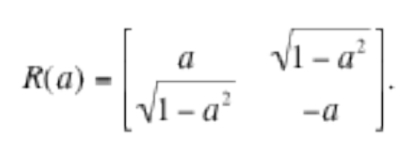In the actual quantum computer, we would observe only what happens to the first qubit (which is being multiplied by a) and ignore everything that happens to the ancillary qubit.

To solve the equation ax = b, then, we need to find a way to implement a unitary transformation with a-1 in the upper left corner. Let's call this transformation U(a):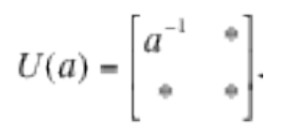The asterisks denote the fact that we do not care very much what numbers are in those locations; they will be whatever they have to be to make U(a) a unitary matrix. Also, notice that U is not just the inverse of R. (In fact, R is its own inverse!)

In 2016, Low (one of Gilyén's three co-authors) discovered an amazing fact that depends purely on classical, nineteenth-century mathematics. If we replace the a-1 in the upper left-hand corner by any polynomial P(a), then we can factor the resulting matrix into a product of unitaries. To be more precise, there exist phase angles ϕ0, ϕ1, …, ϕn that make the following matrix factorization true: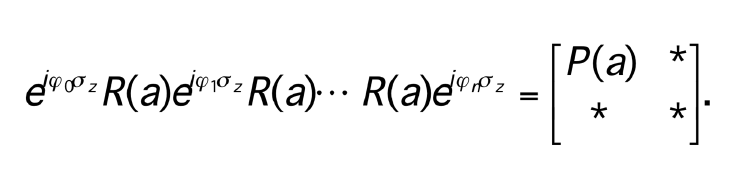Here σz represents the Pauli zed matrix,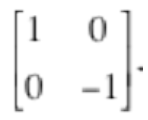Let's also represent the right-hand matrix in this equation by V(a), indicating that it is like our target matrix U(a) but a little bit different.

The significance of this equation is that each operation on the left-hand side can be represented as a quantum gate, specifically a phase shift operator (the ones with the e's) or the operator R(a) that we are given at the outset. Phase shift operators have no analogue on a classical computer; they have to do with the fact that any quantum particle is also a wave, and can be shifted forward or backward by any fraction of a wavelength.

In more conceptual and less mathematical form, we can write the above equation as follows:

V(a) = (phase shift 0) × reflection × (phase shift 1) × … × reflection × (phase shift n).

So the quantum computer can calculate any desired degree-n polynomial P(a) by going through a carefully constructed sequence of (n+1) phase shifts and n repetitions of the process R(a) that gave us the initial data. In all, the computation has a depth of (2n+1) quantum gates.

But the alert reader will object: we don't want to compute P(a)! We want to compute a-1! That observation is correct, but it is possible, again using completely standard classical mathematics, to approximate the function f(a) = a-1 to any desired degree of accuracy (say, error less than ε) on any interval [δ, 1] (where delta and epsilon are thought of as small positive numbers), using a polynomial P(a). There is a price to pay for this approximation: the more accuracy you want, the higher the degree of the polynomial must be, and therefore the larger the number of quantum operations will be. Gilyén and his colleagues showed that the number of operations required is proportional to (|log (1/ε)|/δ.

Again, this may seem harder than simply reaching for your calculator and computing a-1, but it's not. In fact, your classical calculator will also require at least log(1/ε) operations to do this computation to the desired accuracy.

The real quantum speedup will come, though, when we try to solve many equations in many unknowns, or equivalently to solve a matrix equation Ax = b, where A is some m-by-n matrix. The target unitary transformation U(A) now has the following form: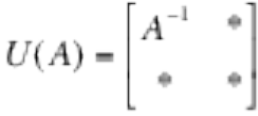,

where A-1 is the inverse (or if A is not square, the pseudo-inverse) of A. If A should happen to be a diagonal matrix (i.e., its only nonzero entries are on the main diagonal), we can do this exactly the same way as before! Each diagonal entry of A-1 is just the reciprocal of the corresponding diagonal entry of A, and we know from above how to compute reciprocals using quantum gates.

Of course, in real life we are seldom so lucky as to be handed a diagonal matrix. What if A isn't a diagonal matrix? In that case, an important theorem in linear algebra comes to the rescue. Any non-diagonal matrix can be brought to diagonal form by pre-multiplying and post-multiplying by two carefully chosen change-of-basis matrices, V and W: that is, VAW is diagonal. This is called the singular-value decomposition of A. (In Low's previous work, he had used a weaker form of diagonalization that requires special assumptions on A. The great virtue of the singular-value decomposition is that it does not require extra assumptions.)

A key point is that the matrices V and W do not increase the quantum complexity of the algorithm. They are used only for analyzing how the algorithm works. "You don't need to go through finding the 2-by-2 matrices," Gilyén says. "Quantum mechanics finds the decomposition for you." In an ideal world, where all gates function perfectly, the quantum computer would be able to approximate A-1 much more quickly than a classical computer.

Remarkably, the same argument can be used to give faster versions of other known quantum algorithms. For instance, fixed point amplitude amplification is a trick that turns a quantum circuit that might get the right answer to a problem into a circuit that will almost certainly get the right answer. Because quantum computers are inherently uncertain, it's important for a quantum programmer to have in his toolkit a technique that, in effect, turns a computer that mimics an "F" student into one that mimics an "A" student.

The trick is this: Suppose that |ψgood〉 is the quantum state that corresponds to the desired solution of your problem, and |ψbad〉 corresponds to the (perhaps many) incorrect solutions. To amplify the correct solution, you first entangle the qubit with an indicator qubit, so the entangled pair is in some superposition of the states |0〉 |ψgood〉 or |1〉 |ψbad〉. Now suppose you are in possession of a quantum algorithm U that ends in state |ψgood〉 at least p percent of the time. When applied to the entangled pair, this algorithm corresponds to a unitary matrix with a number a > √p in its upper left-hand corner: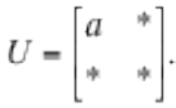By the singular value method of Gilyen and his colleagues, you can then apply a series of quantum gates to this matrix which will transform it into a matrix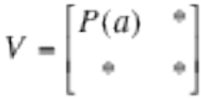,

where P(a) is an arbitrary polynomial. Now the trick is to choose P(a) so that for values of a above the cutoff value √p, the value of the polynomial P(a) is very close to 1. No matter what a is (as long as it is greater than √p), this series of quantum gates will "amplify" the probability of success so that it is very close to 1.

But is it always possible to find a suitable polynomial P(a)? This is again a problem in classical math. The answer is always yes, provided that you are willing to pay a high enough price for it, the price being the degree of the polynomial (which corresponds to the number of gates or operations that the quantum computer needs to do).

The qubitization method described here derives some of its power from the tunability of (ideal) phase shift gates. That is, in principle you can create a phase shift gate with any phase angle ϕ. In effect, a quantum computer un-digitizes the digital computer.

But is this tunability a feature or a bug? Gilyén's group shows that it can be a feature; they use it to perform calculations more efficiently than on a classical computer. But it is also potentially a huge weakness. With continuous gates, every operation will contain a small amount of error. Whether these errors can be kept from adding up and rendering the computation meaningless is still an open question. Computer scientists have developed methods for quantum error correction. However, Gilyén says, "Whether this will work in practice or not will be a fundamental and unprecedented test of the theory of quantum mechanics."

As long as the quantum computers under discussion are only ideal theoretical devices, it makes perfect sense to postpone such questions; theoreticians can always say that they are stockpiling and improving computational tricks for the future. However, the future is moving closer and closer to the present, and imperfect quantum devices may soon become a reality. "The next milestone in quantum computing will be the demonstration of error correcting," Gilyén says. After that will come the first demonstration that a practical quantum device can solve some problem—even a useless one—faster than a classical computer. (See Vignette 3.) After that, says Gilyén, "hopefully we will get to the regime of practical quantum algorithms," — and then techniques like qubitization and quantum singular value transformations will have their day in the sun.

Vignette 2: Quantum Chemistry  The Right Answers for the Right Reasons
Some of the most popular lectures at this summer's Challenges in Quantum Computing cluster had to do with neither quantum physics nor computer science: they were about chemistry. Attendees were eager to learn about what some have called the most promising near-term application of quantum computing: quantum chemistry, or the simulation of chemical reactions using the principles of quantum mechanics.

Of course, the interest goes in both directions: chemists are also interested in quantum computing as a way out of a stalemate they now face. "We have learned how to solve the wrong equations very precisely," said Martin Head-Gordon, a chemist at UC Berkeley. "A perfectly accurate simulation is infeasible, and a perfectly feasible simulation is inaccurate."

Quantum chemistry begins with quantum physics, and in particular with Schrödinger's equation, which describes the evolution of a quantum particle or wave. In chemical applications, the particles will generally be electrons, which are subject to electromagnetic forces from other electrons and from atomic nuclei. In situations of interest to chemistry, there will often be several nuclei present, because chemists are most interested in what happens to the electrons during a chemical reaction between two or more molecules.

A key player in Schrödinger's equation is the Hamiltonian, an operator that represents the total energy in the system. In principle, once you know the Hamiltonian, you can predict what the system will do. In practice, this is not so easy, especially when there are many particles in the system.

In chemical applications, the Hamiltonian H consists of four terms: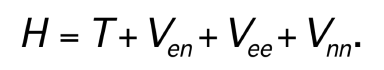The first term, T, represents the kinetic energy of the electron(s). The second, Ven, represents the potential energy due to the electrical attraction between the electrons and the atomic nuclei. The third, Vee, represents the potential energy due to the electrical repulsion between electrons. ("That's the exciting part," says Head-Gordon.) The last term, Vnn, represents the energy due to the electrical repulsion between neighboring nuclei. As far as the electrons are concerned, this term is just a constant; but for the chemist, it should be interpreted more as a parameter in the model. If you put the nuclei in different positions, the internuclear forces will change.

It is helpful to think of the Hamiltonian as defining an energy landscape with peaks and valleys, and passes between the valleys. A chemical reaction will typically pass through several intermediate steps; at each intermediate step, the molecular configuration climbs from one energy minimum up over a pass, and then descends to the energy minimum in the next valley.

It is usually easy for a chemist to identify the energy minima, because they are stable states. Imagine for example, a chair, which has two stable states corresponding to energy minima: it can stand on four legs, or it can lie on the floor. In between those two stable states there is a meta-stable state, when the chair is balancing precariously on two legs instead of four. The chair will only remain in that state for a short period of time, yet that short-lived moment when the chair is standing on two legs is very important. If you want to know how much energy it takes to push the chair over, you have to know only how much energy it takes to reach that meta-stable state; after that, gravity (or energy minimization) will do the rest. In the same way, the rate of a chemical reaction is governed by the height of the energy barrier the system must pass over.

Because the meta-stable configurations are hard to make in the lab, their properties have to be worked out mathematically, by using Schrödinger's equation. Yet, at present, chemists prefer to take what Head-Gordon calls a beautiful sidestep that circumvents the full complications of Schrödinger's equation. This sidestep is called density functional theory, and it essentially treats the electrons in the atom or molecule as a uniform electron gas rather than as a small number of rapidly moving particles. This approximation is usually good enough to give qualitatively correct answers about the intermediate steps of the reaction. Therefore, Head-Gordon says, "95 percent of chemistry is done with density functional theory."

Nevertheless, as James Whitfield of Dartmouth College says, "It gets the right answers for the wrong reasons." And once you start asking quantitative questions, like reaction rates, it doesn't even get the right answers. "It's ideal for classical computers, and we can use it to routinely solve problems for up to 100 atoms, but it does not often achieve chemical accuracy," says Head-Gordon.

Chemists are excited about quantum computing because it may enable them to get a handle on Schrödinger's equation for reasonably complex reactions, without making overly simplistic assumptions as in density functional theory. The idea makes sense: Why not use one quantum system, i.e., a quantum computer, to simulate another?

The types of chemical reactions where this ability will be useful will, of course, be the ones where the electron gas approximation is most inaccurate. These include catalyzed reactions, in which catalysts shuttle electrons back and forth between reactants; reactions involving heavy metals, in which electrons reach relativistic velocities; and especially systems with strong correlations between electrons. These correlations explicitly violate the assumption that the electrons are distributed uniformly. One example cited by Head-Gordon is not a reaction but a single organic molecule called ferrodoxin, whose core consists of four iron atoms and four sulfur atoms arranged in a cube. The electrons in two of the irons are in one spin state and the electrons in the other two are in another. "Now a minimum of 22 electrons in the iron atoms are entangled, and that's not even counting the sulfur atoms, which contribute 24 more electrons!" says Head-Gordon. "Even the low-lying excited states [of this molecule] are beyond the ability of classical computers to predict."

Nobody can be sure yet whether quantum computers will be large enough or accurate enough to do what classical computers cannot. In 2016, though, physicist Nathan Wiebe of Microsoft teamed up with chemist Markus Reiher of ETH in Switzerland to work out a serious ballpark estimate of the power a quantum computer would need. The reaction they chose to simulate is the process of nitrogen fixation by a bacterial enzyme called nitrogenase. In this reaction, the triple bond between the two nitrogen atoms in an N2 molecule is broken, and the nitrogens are incorporated into two ammonia (NH3) molecules. In this way, nitrogen from the atmosphere is converted to a biologically usable form. Legumes and grasses, for example, depend on symbiotic bacteria to perform this task for them.

The energy barrier for this reaction is very high. At present, scientists who want to produce ammonia for fertilizers have to use a catalyst that works only at high temperatures and pressures. "One-and-a-half percent of our global energy consumption is used for nitrogen fixation," says Wiebe. "But a bacterium does it at low temperature. Chemists haven't been able to understand the mechanism yet, but we think it's borderline feasible with quantum computing."

By extrapolating from simulations of simpler reactions, Wiebe and his team estimated the number of qubits and the number of quantum gates (roughly speaking, the number of operations) that would be needed to simulate the nitrogenase reaction: 108 qubits and about 2 quadrillion gates. They conservatively estimated the runtime of such a simulation at 130 days. However, a variety of conventional speedup methods, such as parallelizing the computation, could bring the time down to a few hundred hours.

Because Microsoft and other companies are already talking about building 50-qubit quantum devices, that part of Wiebe's hypothetical calculation does indeed seem "borderline feasible". Implementing that many gates in a fault-tolerant way might take more effort. Roughly half of them have to be T gates, which are similar to the phase shift operators discussed in Vignette 1 but with a fixed phase of π/8. This non-classical phase shift operation roughly corresponds to flipping a bit one-eighth of the way from on to off, or vice versa.

There is, however, one big catch to this estimate of the resources necessary to solve the nitrogenase problem. The 108 qubits would have to be flawless, logical qubits that do not make mistakes. With current technology, however, the error rate of a qubit is roughly one error per 1,000 operations. In a calculation that requires 200 million operations, there will surely be many thousands of errors. Therefore another step is required: error correction. To get the error rate down to an acceptable rate, you would need thousands of physical qubits in place of each logical qubit. (You can think of the physical qubits as "voting" for the answer that the logical qubit should produce; the actual error correcting process is a good deal more complicated.)

Possibly for reasons like this, the companies working on quantum computing hardware seem to be placing more and more emphasis on the reliability of qubits. As John Martinis of Google said, "You don't need just a new gate but a good gate, in fact a damn good gate. Quality is way more important than quantity."

While Wiebe's result shows one direction in which quantum chemistry could go, other participants in the cluster presented other ideas. Andrew Childs reported on his research on a one-dimensional quantum spin system, in which quantum particles are arranged along a line and the Hamiltonian expresses the fact that adjacent particles prefer to have their spins aligned in the same direction. But the Hamiltonian also includes an external magnetic field that varies randomly from place to place, and which gives some of the spins an incentive not to point in the same direction. As Childs asks, "What happens as you crank up the disorder?" He expects to see a phase transition from a thermal state, where local variations in the magnetic field get smoothed out, to a localized state where the fluctuations in the magnetic field leave a fingerprint on the spin system.

While Childs' example falls on the physics side of the divide between physics and chemistry, he argues that a simple system like this one is likely to be simulated successfully sooner than a full-blown chemical model. For example, the interactions between particles are much simpler because each particle only interacts with two neighbors. In a 2017 paper, Childs estimated that a 50-particle spin system could be simulated with a 50-qubit computer using 2 billion T-gates, much less than the number of gates needed for Wiebe's nitrogenase model.

James Whitfield wants to see more research on newer chemical models called coupled cluster models and R12 theory, which consider pairs of electrons as a unit. Coupled cluster models won't replace density functional models for the bulk of applications, but they do work much better for systems with strongly correlated electrons.

"A lot of chemistry is built on one-particle theory because it's easier, but what seemed hard in the 1930s, when computational chemistry was developed, may not be hard any more with quantum computers," Whitfield says. "We have gotten used to a hammer, but if you try to build a house with a hammer you might miss some of the things you can do with a screwdriver. On the other hand, if you have a screwdriver, you still need a hammer to put 95 percent of the house together!"

Vignette 3: Speaking in a Quantum Accent
Over the last four years, large companies, such as IBM, Google and Microsoft, as well as smaller companies, such as Rigetti, have announced their ability or intentions to create systems consisting of moderate numbers of qubits at a time: say, more than 10 but less than 100. Because of the exponential speedup discussed earlier, it is possible to imagine such systems doing computations far beyond the abilities of a classical computer. However, these devices will still be extraordinarily finicky and difficult to control, and very mistake-prone. They are steps in the direction of universal quantum computing, but the finish line is still several laps away.

In view of these limitations, it is natural to ask whether the early quantum machines will be good for anything at all. This is by no means an idle question. So far, the Googles and Microsofts are approaching the problem in the experimental spirit, and not worrying yet about the economic case for building these devices. But they would surely not be investing millions of dollars in quantum computing merely for the sake of a pure physics demonstration. At some point, quantum computers will have to do something more quickly and efficiently than our existing electronic computers. In fact, history suggests that quantum computers will need to be much faster and more efficient if they are to have any hope of displacing the already existing, well-developed technology.

There are some reasons for optimism. For more than 25 years, computer scientists have known in theory that quantum computers can do some things better than classical, or non-quantum computers. But in the absence of any real quantum devices, computer scientists had to approach this question in a manner that was agnostic about the details of how a quantum computer would work. Peter Shor's algorithm for factorization of integers, announced in 1994, was the most dramatic example, because the problem is so important and the speedup is so dramatic. Regardless of the details of how fast the quantum gates work, for example, for large enough integers, Shor's algorithm will be faster than any algorithm known on a classical computer. There are other algorithms that run faster in theory on a quantum computer, such as Grover's algorithm for search, but their margin of superiority is not so dramatic. If the quantum computer's gate speed is too slow, the theoretical advantage might not be realized at all.

Even Shor's algorithm runs into another issue: it assumes that the quantum gates operate without errors. In reality that assumption will not be true, and it will need an additional layer of error correction. Thus, Shor's algorithm will require hundreds of thousands of physical qubits to achieve the necessary accuracy. That puts it well beyond the range of the systems under development. Some other algorithm (something much more error-tolerant) will be needed if computer scientists wish to demonstrate the supremacy of a 50-qubit computer. Even if the particular algorithm has no known useful application, the company that first demonstrates this empirical kind of supremacy will at least derive some sort of bragging rights, which might give it an inside track on controlling the market for quantum computers.

Google's research group on quantum computing has stated its intention to perform an experiment that is very much in this spirit, and could empirically demonstrate quantum supremacy for the first time. They will simply ask their device to simulate itself—or more precisely, to simulate a random quantum circuit. Just as the easiest role for an actor to play is himself (or herself), it ought to be easy for a quantum computer to behave like a quantum device.

But to show that the quantum computer actually has an advantage, you need more than this: you need to show that a classical computer cannot simulate a random quantum circuit, at least in a reasonable amount of time. That is exactly the problem that Adam Bouland, Bill Fefferman, Chinmay Nirkhe, and their advisor Umesh Vazirani solved (subject to some assumptions, discussed below) this spring.

Random is Hard
In one sense, the Google experiment is the antithesis of what quantum computing is supposed to be about. Instead of designing a circuit intelligently to perform a specified task, the Google team will take the monkeys-typing-Shakespeare approach and build random circuits, which most of the time will produce gibberish. However, not all gibberish is the same. If you imagine producing a long string of random syllables in English, it may not sound like Shakespeare, but it will sound recognizably different from a long string of random syllables in German or Chinese. In just the same way, the team of four researchers showed that the Google experiment will produce random noise with a quantum accent. It is precisely the ability to speak in a quantum accent—even while speaking complete nonsense—that cannot be simulated by any classical computer.

It may seem slightly tautological to prove that a quantum computer is better at simulating a quantum computer than a classical computer would be. But as Scott Aaronson of the University of Texas has pointed out, this is exactly where we should expect quantum supremacy to show up first. He compares it to the problem of determining whether dolphins are intelligent. We can do this by subjecting them to a variety of un-dolphin-like tasks, such as jumping through hoops or factoring integers. But it is easier to observe intelligent behavior simply by watching dolphins be dolphins.

Aaronson in fact invoked this argument to motivate the first quantum supremacy proposal, which was called random boson sampling. Some experimental groups have already tried Aaronson's proposed experiment, which involves measurements on photons, but it has proven difficult to implement. Also, says Fefferman, "the optical system is not as clearly on the path to quantum computing." By contrast, building a random quantum circuit is clearly on the road to building controlled quantum circuits, and is thus a reasonable target for a research group like Google's to shoot for.

According to Fefferman and Bouland, verifying quantum supremacy for the random circuit problem involves two steps: first, to show that producing a random output with a "quantum accent" is indeed hard for a classical computer; second, to show that the quantum computer is producing that kind of output. They achieved the first part of the two-step process by a worst-to-average-case reduction—in other words, showing that if we can simulate the average quantum circuit, then we can simulate the worst (most non-classical) one. The probability of observing a given quantum circuit in a given state was already known to be hard to compute in the worst case, and their argument shows that it must therefore also be hard (for a classical computer) in the average case.

The second part of their work—to verify that the quantum device is actually speaking with a quantum accent—is a little bit trickier, and not fully resolved yet. It relies on the fact that the quantum devices in question have only 50 qubits. Although a quantum accent is hard for a classical computer to mimic if the random quantum circuits are large, an intermediate-scale quantum circuit can still be mimicked by a classical computer if the latter is large enough and fast enough. A quantum circuit with 50 qubits (the size that Google is proposing to experiment with) can be mimicked by the world's fastest supercomputers using trillions of classical bits. So in theory, we can use a classical supercomputer to speak with the same quantum accent as a 50-qubit quantum machine. It would not be possible, however, to simulate the accent of a 100-qubit quantum machine. "We're trying not to bite off more than we can chew," observes Fefferman.

But even if we have programmed a classical supercomputer to speak with a quantum accent, how does it recognize a native speaker? What criterion does it use to give the quantum device a passing grade? Because the random quantum circuit is speaking nonsense syllables, there is no such thing as a "right answer," and no way to definitively say that a given output could only be produced by a quantum machine. The only way to verify its output is by a statistical test. The observed quantum nonsense should match the simulated quantum nonsense to a high degree of accuracy, a high percentage of the time. The Google researchers are proposing to measure the degree of matching by a metric called cross-entropy difference. A cross-entropy score of 1 would be an ideal match, and they believe that they can get a score above 0.1.

Fefferman and Bouland give this method a qualified thumbs-up. That is, the cross-entropy score will certify the authenticity of the quantum accent, provided that the alleged quantum device is behaving like an ideal quantum circuit with random noise added, and assuming that the noise always adds entropy to the output.

This point may sound like a tautology, because high entropy is often taken as a synonym for randomness. But the real story is not so simple. Certain kinds of processes that look random can actually reduce entropy. For example, an erasure process that randomly chooses some bits and turns them into zeroes will reduce the entropy, because a string with (say) two 0's for every 1 will by definition have lower entropy than a string with equal numbers of 0's and 1's. So if Google's "random circuits" are generated by a process that allows random erasures, then the cross-entropy would not be a valid measure of quantum supremacy. For this reason, among others, it will be very important for Google to pin down in what ways its device deviates from a real random quantum circuit. During the workshop in the second week of June, John Martinis of the Google team acknowledged that this is a high priority for them: "We really have to understand our error model."

Another issue is that Fefferman and Bouland's group has only proved that simulating a perfect quantum accent is hard for a classical computer. It is still not known whether simulating an accent well enough to get an entropy score of 0.1 is a classically hard problem. "This paper nicely fills a hole in our picture of the complexity of random circuit sampling, by showing that exactly calibrating the output probabilities of such circuits is #P-hard on average," wrote Scott Aaronson in his blog. "The real dream would be to show that even approximating the output probabilities [e.g., getting an entropy score of 0.1] is #P-hard on average." Nobody has done that yet for this or any other proposed random-sampling test of quantum supremacy.

Even if some uncertainties remain, the Google experiment could still provide very important information. "There are actually some severe doubters, who think that errors will always accumulate and prohibit you from demonstrating a large-scale quantum computer," Fefferman says. A sufficiently strong positive result from the Google experiment might not silence the skeptics, but it would at least give them a very narrow ledge to stand on.

,